• 非线性规划（一）：定义与数值优化...非线性规划（二）: Matlab 求解约束极值问题 目录 约束极值问题 1 二次规划 2 罚函数法 3 Matlab 求约束极值问题 3.1 fminbnd 函数 3.2 fseminf 函数 3.3 fminimax 函数...
非线性规划（一）：定义与数值优化方法(梯度法、牛顿法、拟牛顿法、变尺度法)

非线性规划（二）: Matlab 求解约束极值问题

目录

约束极值问题

1  二次规划         2  罚函数法

3  Matlab 求约束极值问题

3.1  fminbnd 函数           3.2  fseminf 函数                 3.3  fminimax 函数

4 Matlab 优化工具箱的用户图形界面解法

4  非线性规划的应用：飞行管理问题

4.1  模型一            4.2  模型二                习 题

约束极值问题

带有约束条件的极值问题称为约束极值问题，也叫规划问题。 求解约束极值问题要比求解无约束极值问题困难得多。为了简化其优化工作，可采用以下方法：将约束问题化为无约束问题；将非线性规划问题化为线性规划问题，以及 能将复杂问题变换为较简单问题的其它方法。 库恩—塔克条件是非线性规划领域中重要的理论成果之一，是确定某点为优点 的必要条件，但一般说它并不是充分条件（对于凸规划，它既是优点存在的必要条件， 同时也是充分条件）。

无约束问题的求解请参考这里：非线性规划（一）：定义与数值优化方法

1  二次规划

若某非线性规划的目标函数为自变量 x的二次函数，约束条件又全是线性的，就称 这种规划为二次规划。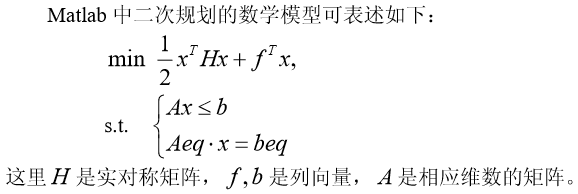Matlab 中求解二次规划的命令是

[X,FVAL]= QUADPROG(H,f,A,b,Aeq,beq,LB,UB,X0,OPTIONS) 

返回值 X 是决策向量 x的值，返回值 FVAL 是目标函数在 x处的值。（具体细节可以参 看在 Matlab 指令中运行 help quadprog 后的帮助）。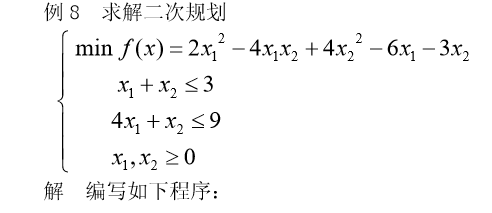h=[4,-4;-4,8];
f=[-6;-3];
a=[1,1;4,1];
b=[3;9];
[x,value]=quadprog(h,f,a,b,[],[],zeros(2,1))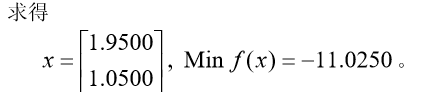2  罚函数法

利用罚函数法，可将非线性规划问题的求解，转化为求解一系列无约束极值问题， 因而也称这种方法为序列无约束小化技术，简记为 SUMT (Sequential Unconstrained Minization Technique)。 罚函数法求解非线性规划问题的思想是，利用问题中的约束函数作出适当的罚函 数，由此构造出带参数的增广目标函数，把问题转化为无约束非线性规划问题。主要有 两种形式，一种叫外罚函数法，另一种叫内罚函数法，下面介绍外罚函数法。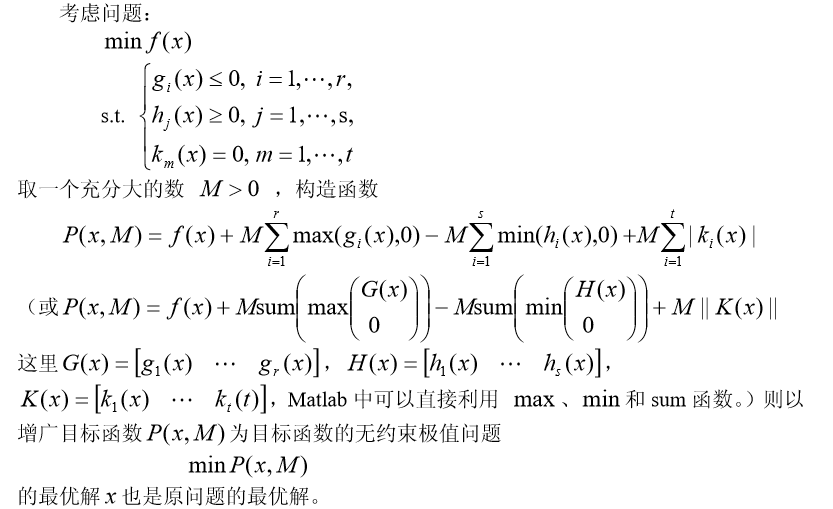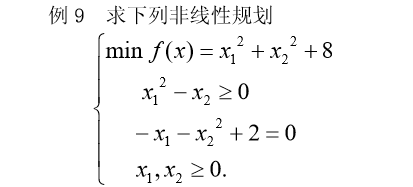解  (i)编写 M 文件 test.m

function g=test(x);
M=50000;
f=x(1)^2+x(2)^2+8;
g=f-M*min(x(1),0)-M*min(x(2),0)-M*min(x(1)^2-x(2),0)+...
M*abs(-x(1)-x(2)^2+2); 

或者是利用Matlab的求矩阵的极小值和极大值函数编写test.m如下：

function g=test(x);
M=50000;
f=x(1)^2+x(2)^2+8;
g=f-M*sum(min([x';zeros(1,2)]))-M*min(x(1)^2-x(2),0)+...
M*abs(-x(1)-x(2)^2+2); 

我们也可以修改罚函数的定义，编写test.m如下：

function g=test(x);
M=50000;
f=x(1)^2+x(2)^2+8;
g=f-M*min(min(x),0)-M*min(x(1)^2-x(2),0)+M*(-x(1)-x(2)^2+2)^2; 

(ii)在 Matlab 命令窗口输入

[x,y]=fminunc('test',rand(2,1)) 

即可求得问题的解。

3  Matlab 求约束极值问题

3.1  fminbnd 函数

求单变量非线性函数在区间上的极小值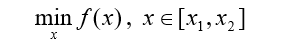Matlab 的命令为

[X,FVAL] = FMINBND(FUN,x1,x2,OPTIONS)

它的返回值是极小点x 和函数的极小值。这里 fun 是用 M 文件定义的函数或 Matlab 中 的单变量数学函数。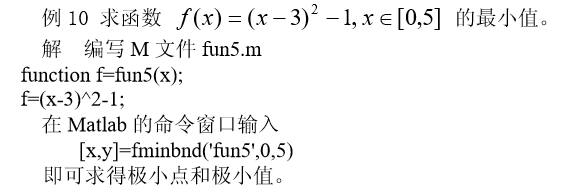3.2  fseminf 函数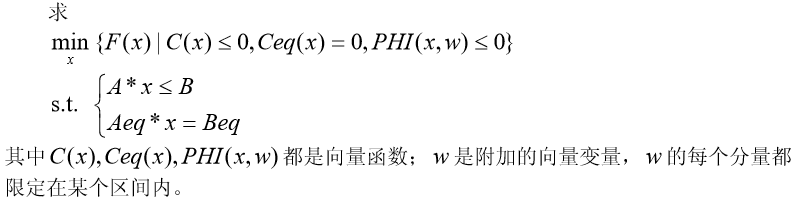上述问题的 Matlab 命令格式为

X=FSEMINF(FUN,X0,NTHETA,SEMINFCON,A,B,Aeq,Beq)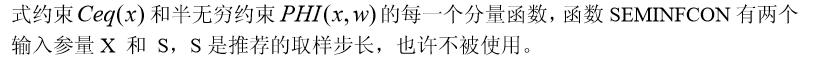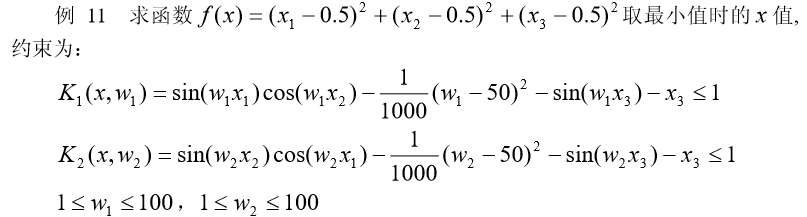解  （1）编写 M 文件 fun6.m 定义目标函数如下：

function f=fun6(x,s);
f=sum((x-0.5).^2); 

（2）编写 M 文件 fun7.m 定义约束条件如下：

function [c,ceq,k1,k2,s]=fun7(x,s);
c=[];ceq=[];
if isnan(s(1,1))
s=[0.2,0;0.2 0];
end
%取样值
w1=1:s(1,1):100;
w2=1:s(2,1):100;
%半无穷约束
k1=sin(w1*x(1)).*cos(w1*x(2))-1/1000*(w1-50).^2-sin(w1*x(3))-x(3)-1; k2=sin(w2*x(2)).*cos(w2*x(1))-1/1000*(w2-50).^2-sin(w2*x(3))-x(3)-1;
%画出半无穷约束的图形
plot(w1,k1,'-',w2,k2,'+'); 

（3）调用函数 fseminf 在 Matlab 的命令窗口输入

[x,y]=fseminf(@fun6,rand(3,1),2,@fun7) 

即可求得结果。

3.3  fminimax 函数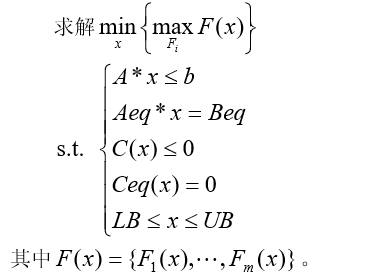上述问题的 Matlab 命令为

 X=FMINIMAX(FUN,X0,A,B,Aeq,Beq,LB,UB,NONLCON)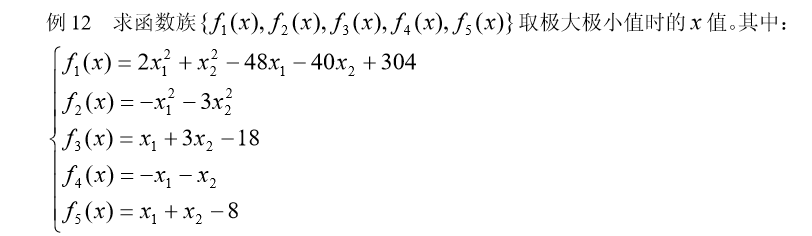解  （1）编写 M 文件 fun8.m 定义向量函数如下：

function f=fun8(x);
f=[2*x(1)^2+x(2)^2-48*x(1)-40*x(2)+304
-x(1)^2-3*x(2)^2
x(1)+3*x(2)-18
-x(1)-x(2)
x(1)+x(2)-8]; 

（2）调用函数 fminimax

[x,y]=fminimax(@fun8,rand(2,1)) 

3.3.4  利用梯度求解约束优化问题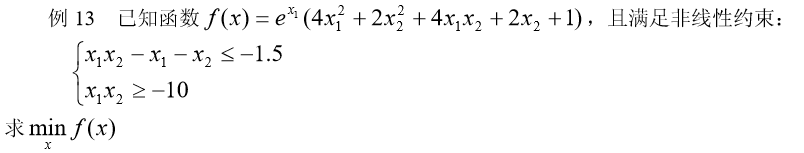分析：当使用梯度求解上述问题时，效率更高并且结果更准确。 题目中目标函数的梯度为：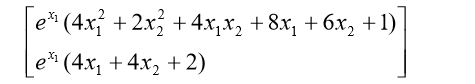解  （1）编写 M 文件 fun9.m 定义目标函数及梯度函数：

function [f,df]=fun9(x);
f=exp(x(1))*(4*x(1)^2+2*x(2)^2+4*x(1)*x(2)+2*x(2)+1);
df=[exp(x(1))*(4*x(1)^2+2*x(2)^2+4*x(1)*x(2)+8*x(1)+6*x(2)+1);exp(x(1))*(4*x(2) +4*x(1)+2)]; 

（2）编写 M 文件 fun10.m 定义约束条件及约束条件的梯度函数：

function [c,ceq,dc,dceq]=fun10(x);
c=[x(1)*x(2)-x(1)-x(2)+1.5;-x(1)*x(2)-10];
dc=[x(2)-1,-x(2);x(1)-1,-x(1)]; ceq=[];dceq=[]; 

（3）调用函数 fmincon，编写主函数文件 example13.m 如下：

%采用标准算法
options=optimset('largescale','off');
%采用梯度
[x,y]=fmincon(@fun9,rand(2,1),[],[],[],[],[],[],@fun10,options)


4 Matlab 优化工具箱的用户图形界面解法

Matlab 优化工具箱中的 optimtool 命令提供了优化问题的用户图形界面解法。 optimtool 可应用到所有优化问题的求解，计算结果可以输出到 Matlab 工作空间中。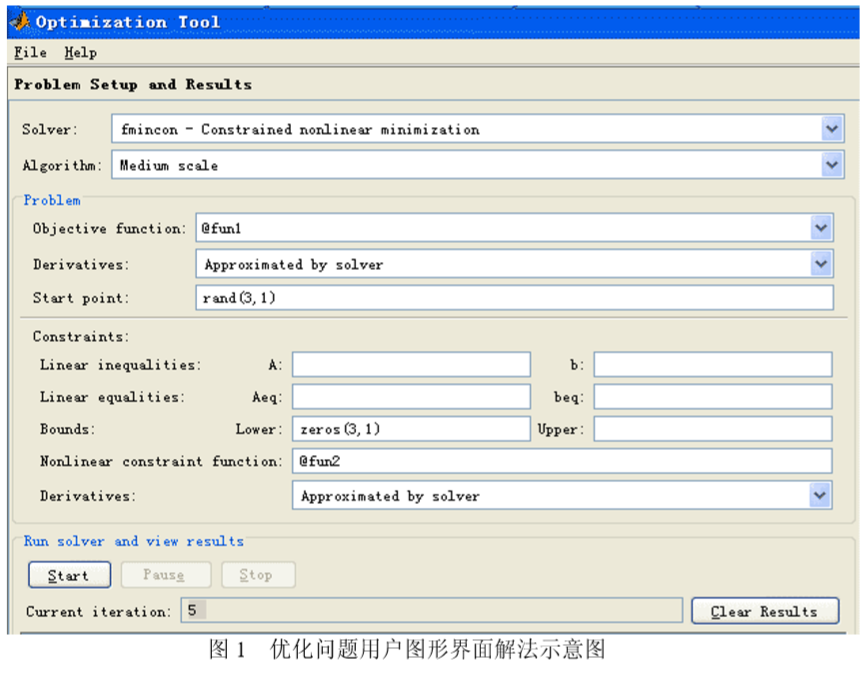例 14  用 optimtool 重新求解例 2。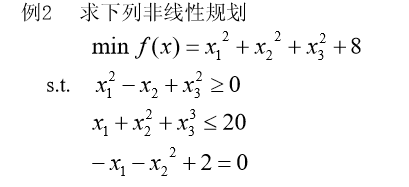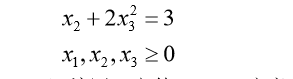解  （i）编写 M 文件 fun1.m 定义目标函数

function f=fun1(x);
f=sum(x.^2)+8;


（ii）编写M文件fun2.m定义非线性约束条件

function [g,h]=fun2(x);
g=[-x(1)^2+x(2)-x(3)^2
x(1)+x(2)^2+x(3)^3-20];  %非线性不等式约束
h=[-x(1)-x(2)^2+2
x(2)+2*x(3)^2-3]; %非线性等式约束 

利用例 2 已经定义好的函数 fun1 和 fun2。在 Matlab 命令窗口运行 optimtool，就打 开图形界面，如图 1 所示，填入有关的参数，未填入的参数取值为空或者为默认值，然 后用鼠标点一下“start”按钮，就得到求解结果，再使用“file”菜单下的“Export to Workspace…”选项，把计算结果输出到 Matlab 工作空间中去。

4  非线性规划的应用：飞行管理问题

在约 10，000m 高空的某边长 160km 的正方形区域内，经常有若干架飞机作水平 飞行。区域内每架飞机的位置和速度向量均由计算机记录其数据，以便进行飞行管理。 当一架欲进入该区域的飞机到达区域边缘时，记录其数据后，要立即计算并判断是否会 与区域内的飞机发生碰撞。如果会碰撞，则应计算如何调整各架（包括新进入的）飞机 飞行的方向角，以避免碰撞。现假定条件如下：

1）不碰撞的标准为任意两架飞机的距离大于 8km;

2）飞机飞行方向角调整的幅度不应超过 30 度；

3）所有飞机飞行速度均为每小时 800km;

4）进入该区域的飞机在到达区域边缘时，与区域内飞机的距离应在 60km 以上；

5）多需考虑 6 架飞机；

6）不必考虑飞机离开此区域后的状况。

请你对这个避免碰撞的飞行管理问题建立数学模型，列出计算步骤，对以下数据进 行计算（方向角误差不超过 0.01 度），要求飞机飞行方向角调整的幅度尽量小。

设该区域 4 个顶点的座标为(0,0)，(160,0)，(160,160)，(0,160)。记录数据见表 1。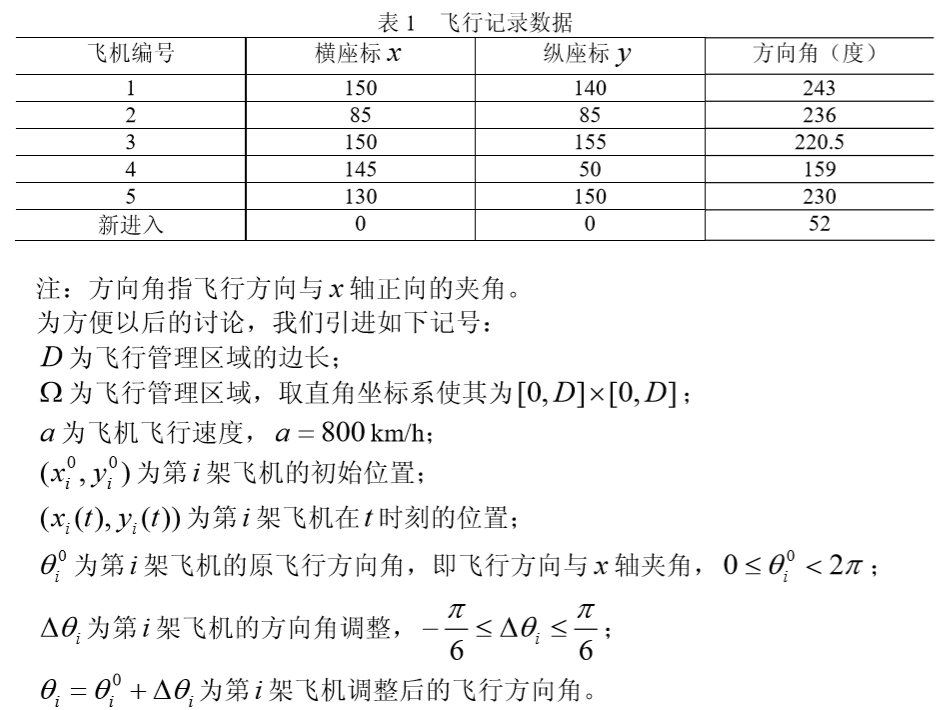4.1  模型一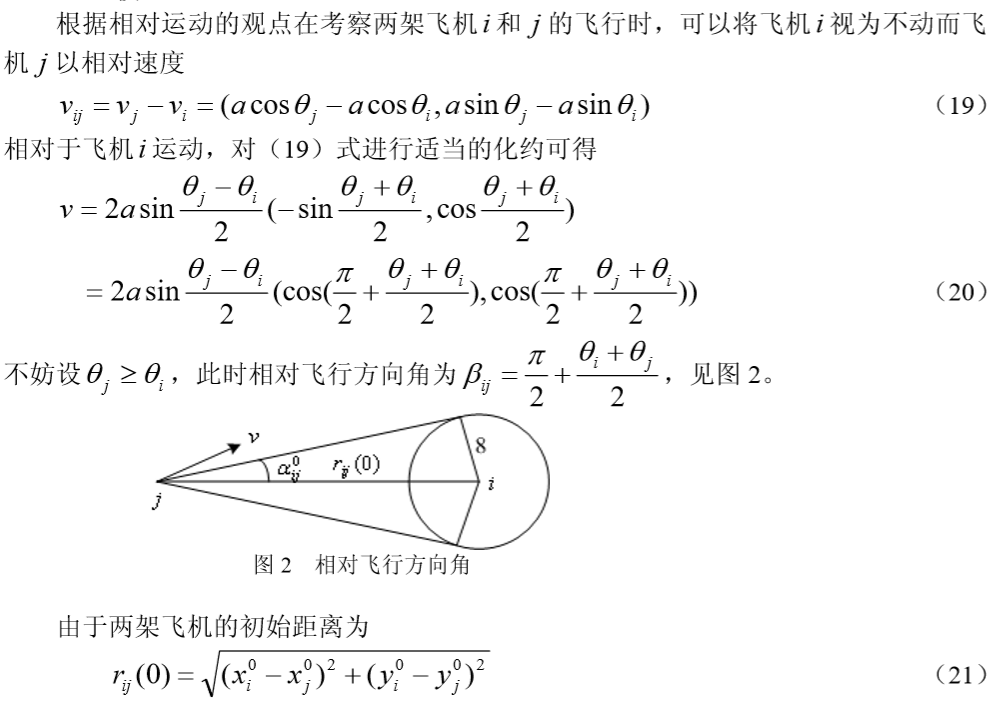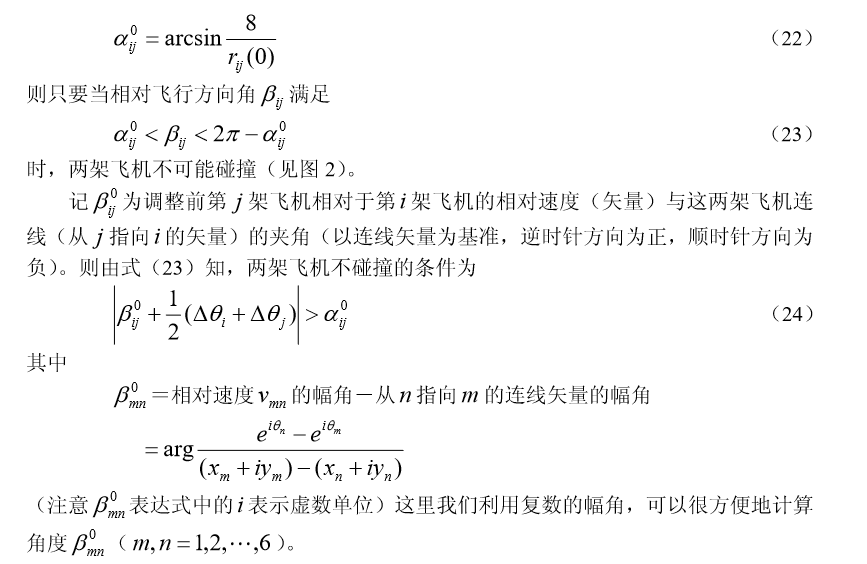本问题中的优化目标函数可以有不同的形式：如使所有飞机的大调整量小； 所有飞机的调整量绝对值之和小等。这里以所有飞机的调整量绝对值之和小为目标 函数，可以得到如下的数学规划模型：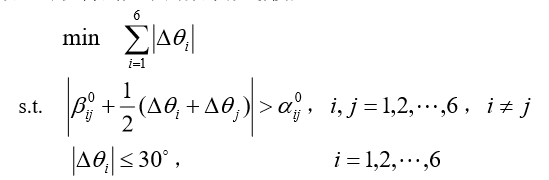利用如下的程序：

clc,clear
x0=[150 85 150 145 130 0];
y0=[140 85 155 50 150 0];
q=[243 236 220.5 159 230 52];
xy0=[x0; y0];
d0=dist(xy0);   %求矩阵各个列向量之间的距离
d0(find(d0==0))=inf;
a0=asind(8./d0)  %以度为单位的反函数
xy1=x0+i*y0 xy2=exp(i*q*pi/180)
for m=1:6
for n=1:6
if n~=m
b0(m,n)=angle((xy2(n)-xy2(m))/(xy1(m)-xy1(n)));
end
end
end
b0=b0*180/pi;
dlmwrite('txt1.txt',a0,'delimiter', '\t','newline','PC');
fid=fopen('txt1.txt','a');
fwrite(fid,'~','char');       %往纯文本文件中写 LINGO 数据的分割符 dlmwrite('txt1.txt',b0,'delimiter', '\t','newline','PC','-append','roffset', 1)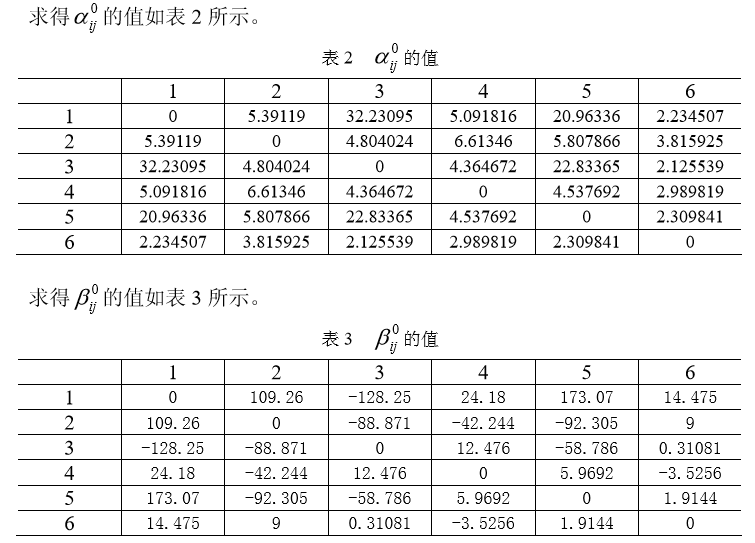上述飞行管理的数学规划模型可如下输入 LINGO 求解：

model:
sets:
plane/1..6/:delta;
endsets
data:
alpha=@file('txt1.txt');   !需要在alpha的数据后面加上分隔符"~";
beta=@file('txt1.txt');
enddata
min=@sum(plane:@abs(delta));
lpha(i,j));
end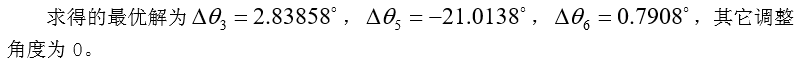4.2  模型二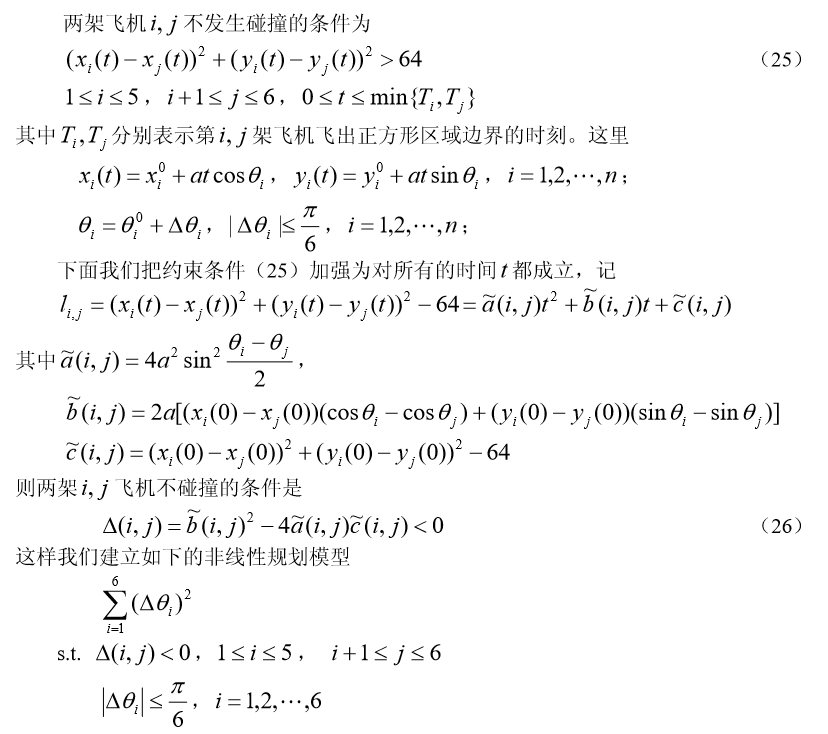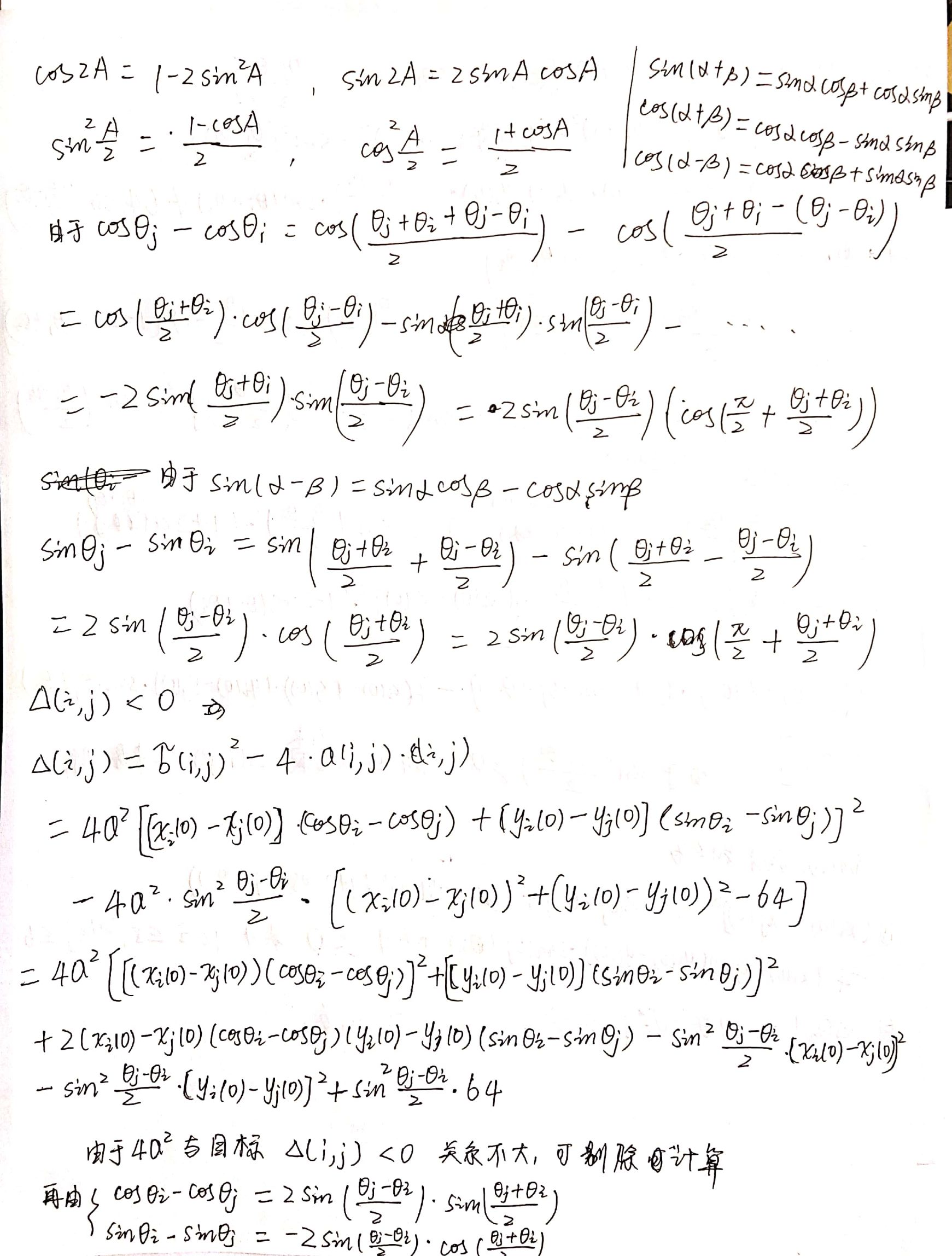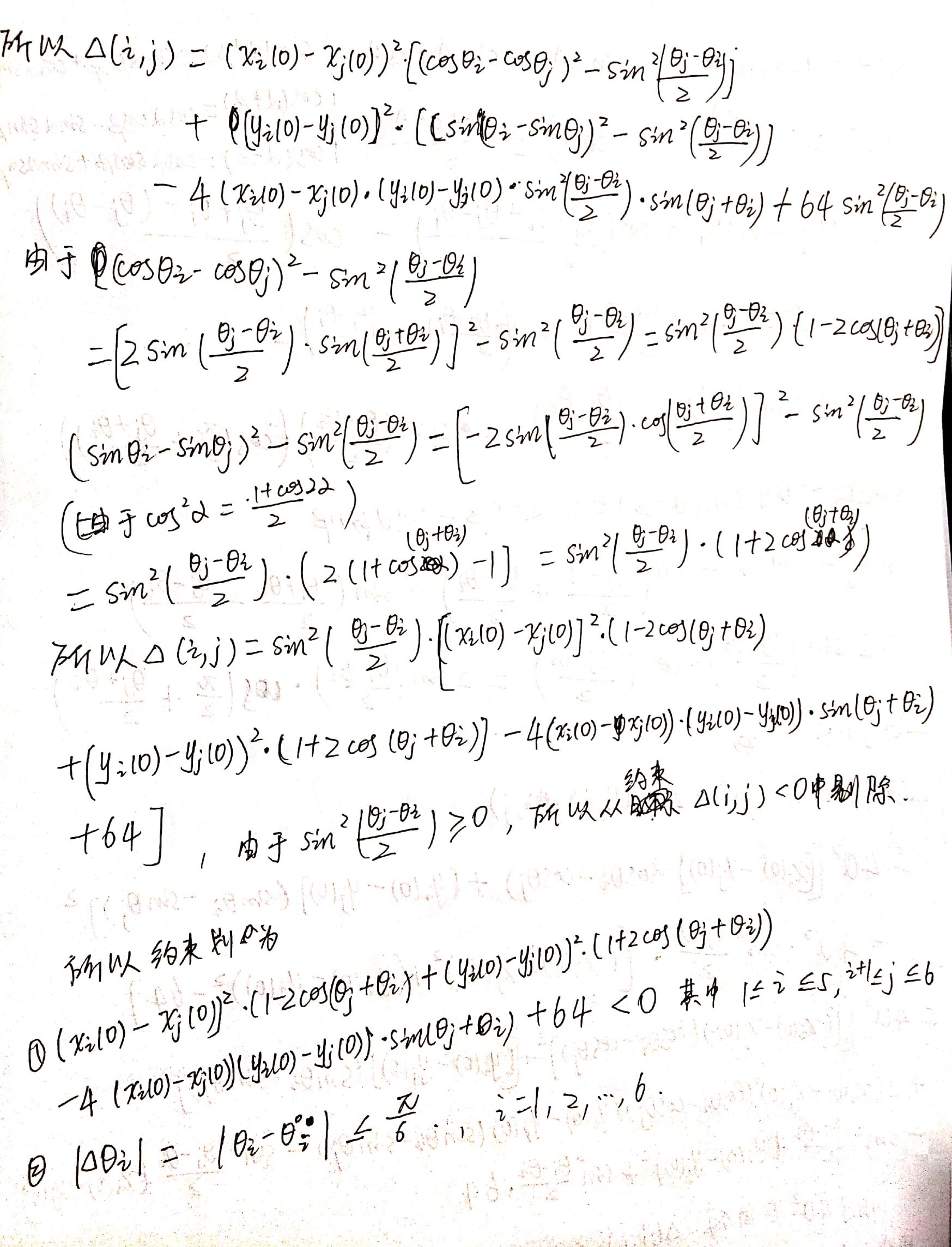计算到这里也没有展开的必要了。由于目标的约束是< 0和 <= 的不等式约束。用罚函数的方式编写和求解，有思路的人请留言到评论区讨论吧

习 题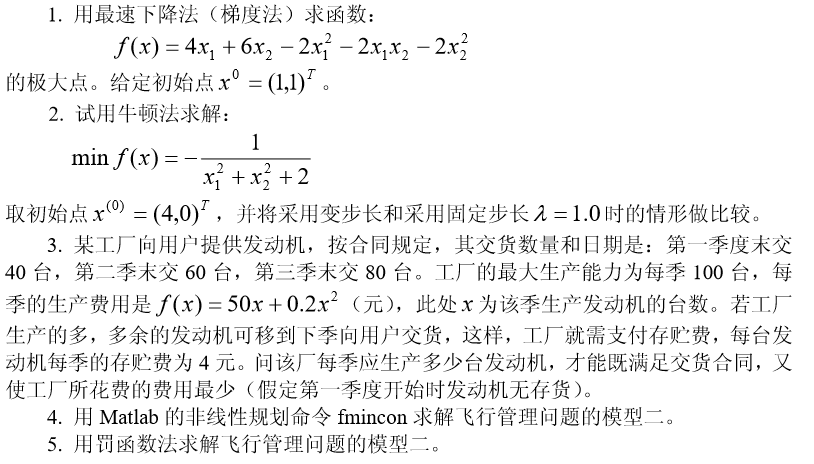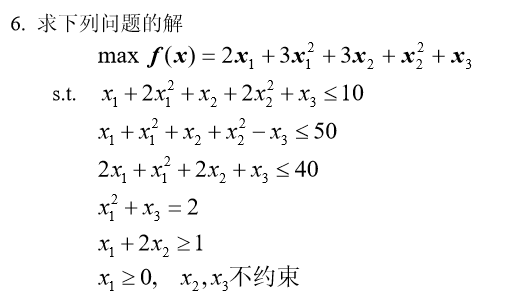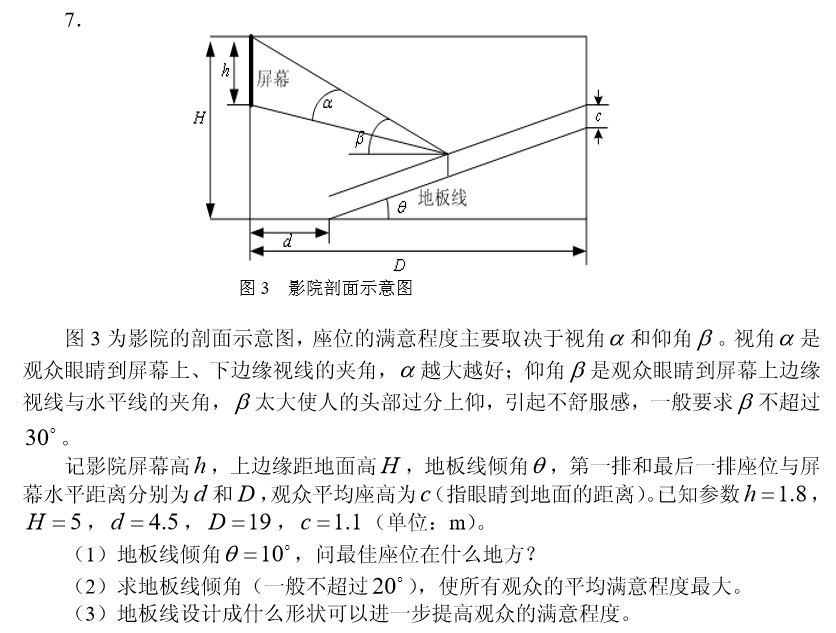非线性规划（一）：定义与数值优化方法(梯度法、牛顿法、拟牛顿法、变尺度法)

非线性规划（二）: Matlab 求解约束极值问题

展开全文• 约束极值问题的定义及优化方法 带有约束条件的极值问题称为约束极值问题，也叫规划问题。 求解约束极值问题要比求解无约束极值问题困难得多。为了简化其优化工作，可采用下面两种方法: 1.将约束问题转化为无约束...

约束极值问题的定义及优化方法

带有约束条件的极值问题称为约束极值问题，也叫规划问题。
求解约束极值问题要比求解无约束极值问题困难得多。为了简化其优化工作，可采用下面两种方法:
1.将约束问题转化为无约束问题；
2.将非线性规划问题转化为线性规划问题。

什么样的目标函数可以用二次规划求解呢？
1.非线性规划的目标函数为自变量x的二次函数
2.约束条件是线性的

二次规划数学模型其中，H是实对称矩阵；f,b,beq,lb,ub是列向量；A,Aeq是相应维数的矩阵。
Matlab中求解二次规划的命令：
返回值x是决策向量x的值，返回值fval是目标函数在x处的值。

二次规划求解的例子

Example：编写程序如下：
H=[4,-4;-4,8];
f=[-6;-3];
A=[1,1;4,1];
b=[3,9];
这里x1和x2有下界，没有上界，所以根据第三个约束，lb为两行一列的0矩阵，上界为空。
求解得：x1=1.9500，x2=1.0500，minf（x）=-11.0250


展开全文二次规划
• 最后修改日期 2015/11/7无约束极值问题可以表述为 minf(X),X∈Rnmin f(X), X \in R^n 对于这类问题的求解一般要用到迭代法。迭代法可分为两大类。一类是要用到函数一阶导数或二阶导数的解析法；另一类是迭代过程中...
华电北风吹
最后修改日期 2015/11/7

无约束极值问题可以表述为 minf(X),X∈Rn<!--//--><![CDATA[//><!--
min f(X), X \in R^n
//--><!]]>
对于这类问题的求解一般要用到迭代法。迭代法可分为两大类。一类是要用到函数一阶导数或二阶导数的解析法；另一类是迭代过程中只用到函数值的直接法。常见的解析法有梯度下降法，共轭梯度法，变尺度法。常见的直接法有步长加速法。

一、梯度下降法
假设Xk<!--//--><![CDATA[//><!--
X^{k}
//--><!]]>表示极小值点的第k次迭代。求解第k+1次迭代过程为 Xk+1=Xk+λPk<!--//--><![CDATA[//><!--
X^{k+1}=X^{k}+\lambda P^k
//--><!]]>Pk<!--//--><![CDATA[//><!--
P^k
//--><!]]>表示函数f(X)<!--//--><![CDATA[//><!--
f(X)
//--><!]]>在点Xk<!--//--><![CDATA[//><!--
X^k
//--><!]]>处的负梯度。λ<!--//--><![CDATA[//><!--
\lambda
//--><!]]>是步长。
其中，步长可以设定为一个常数值。当然，也可以通过一维搜索求解使得函数f(X)<!--//--><![CDATA[//><!--
f(X)
//--><!]]>在点Xk<!--//--><![CDATA[//><!--
X^k
//--><!]]>沿方向Pk<!--//--><![CDATA[//><!--
P^k
//--><!]]>下降最多的步长(最速下降法)。

二、共轭梯度法

三、变尺度法

四、步长加速法
展开全文this.p={  m:2,b:2,loftPermalink:'',id:'fks_087066084083086074082084095068072084084066087087095066082',blogTitle:'有两个约束条件的条件极值',blogAbstract:'本博文推导三元函数在两个约束条件下取得极值的必要条件。我们使用的教材(同济大学《高等数学》第六版)给出了有两个约束条件的条件极值的拉格朗日乘数法，但是没有给出推导。现将推导补充于下，供大家参考。',blogTag:'',blogUrl:'blog/static/251319162012230105322207',isPublished:1,istop:false,type:0,modifyTime:1333367824564,publishTime:1333076077913,permalink:'blog/static/251319162012230105322207',commentCount:0,mainCommentCount:0,recommendCount:0,bsrk:-100,publisherId:0,recomBlogHome:false,currentRecomBlog:false,attachmentsFileIds:[],vote:{},groupInfo:{},friendstatus:'none',followstatus:'unFollow',pubSucc:'',visitorProvince:'',visitorCity:'',visitorNewUser:false,postAddInfo:{},mset:'000',mcon:'',srk:-100,remindgoodnightblog:false,isBlackVisitor:false,isShowYodaoAd:true,hostIntro:'',hmcon:'1',selfRecomBlogCount:'0',lofter_single:''}{list a as x}{if !!x}{if x.visitorName==visitor.userName}{else}{/if}{if x.moveFrom=='wap'}{elseif x.moveFrom=='iphone'}{elseif x.moveFrom=='android'}{elseif x.moveFrom=='mobile'}{/if}${fn(x.visitorNickname,8)|escape}{/if}{/list}{if !!a}${fn(a.nickname,8)|escape}${a.selfIntro|escape}{if great260}${suplement}{/if}{/if}{list a as x}{if !!x}推荐过这篇日志的人：{list a as x}{if !!x}{if !!b&&b.length>0}他们还推荐了：{list b as y}{if !!y}{/if}{list a as x}{if !!x}{list a as x}{if !!x}{list a as x}{if !!x}{list a as x}{if x_index>4}{break}{/if}{if !!x}${fn1(x.title,60)|escape}${fn2(x.publishTime,'yyyy-MM-dd HH:mm:ss')}{/if}{/list}{list a as x}{if !!x}{if !!(blogDetail.preBlogPermalink)}网易新闻${headlines.title|escape}{if defined('newslist')&&newslist.length>0}{list newslist as x}{if x_index>7}{break}{/if}被推荐日志最新日志该作者的其他文章博主推荐随机阅读首页推荐{list a as x}{if !!x}${x.nickName|escape}  投票给{var first_option = true;}{list x.voteDetailList as voteToOption}{if voteToOption==1}{if first_option==false},{/if}  “${b[voteToOption_index]}”{/if}{/list}{if (x.role!="-1") },“我是${c[x.role]}”  {/if}\${fn1(x.voteTime)}{if x.userName==''}{/if}{/if}{/list}
展开全文• 等式约束极值、拉格朗日乘子法、不等式约束极值、KT条件KKT条件 拉格朗日函数
• 2019独角兽企业重金招聘Python工程师标准>>> ...
• 设某约束极值问题中的目标函数为 f ( x ) f(x) ，约束条件为 g i ( x ) ≥ 0 g_i(x)\ge 0 ， h i ( x ) = 0 h_i(x)=0 1.非线性规划的一般形式 ⎧ ⎩ ⎨ m i n f ( x ) h i ( x ) = 0 , g i ( x ) ≥ 0 , i = ...非线性
• 1、消元法通过等式约束条件消去一个变量，得到其他变量关于该变量的表达式代入目标函数，转化为无约束的极值求解问题，具体过程如下：得到无约束极值问题即可通过：一阶导数=0求驻点，Hession矩阵判定极值点。...
• 2019独角兽企业重金招聘Python工程师标准>>> ...
• 2019独角兽企业重金招聘Python工程师标准>>> ...
• 2019独角兽企业重金招聘Python工程师标准>>> ...
• 2019独角兽企业重金招聘Python工程师标准>>> ...起作用约束和可行下降方向的概念 库恩 - 塔克条件   转载于:https://my.oschina.net/liyangke/blog/2984846
• 第一类： 无约束最优化问题找到一个合适的x，是的f(x)最小： minxf(x) \min_x f(x) 没有任何约束的最优化问题，这个一般解法有 梯度下降法、牛顿法、拟牛顿法等。第二类： 有等式约束的非线性minxf(x)subject to hi...非线性优化 拉格朗日乘子法
•一元函数 多元函数
• 畸形约束极值点优化问题的经典优化方法对比，李春明，，对于边界与目标函数等值线接近于相切的畸形约束极值点优化问题，对比了随机方向方法、复合形法、内点惩罚函数法、外点惩罚函数法
• 基础知识预备(1)无约束极值 求y的极值，对函数求导 ，令导数等于0，则x=0就是它的极值点y=0。同时，对 绘制图形为U形曲线，找到这个曲线...简单来说，无约束极值问题，可以通过求导(前提是可以求导)来找到极值。(2)...
• 多元函数极值问题 可以分为以下三个方面 无约束极值问题 等式约束条件极值问题 不等式约束条件极值问题 无约束条件的多元函数极值算法
• 内含有matlab关于求解无约束多维极值问题的所有m函数文件及使用说明。
• 拉格朗日乘数法是用来求条件极值的，极值问题有两类，其一，求函数在给定区间上的极值，对自变量 没有其它要求，这种极值称为无条件极值。其二，对自变量有一些附加的约束条件限制下的极值，称为 条件极值。例如...
• matlab无约束一维极值问题的matlab代码及有关编程
• 内含有matlab关于求解无约束一维极值问题的所有m函数文件及使用说明。
• 约束一维极值问题   极值问题表达式：min f(x) x,x[ x1 x2];   一维极值的搜索方式包括线性搜索和非线性搜索，线性搜索包含黄金分割法、斐波那契法和牛顿法，非线性方法包含抛物线法和三次样条插值。 ...
• 可以实现，进退法。进退法是用来确定搜索区间的算法。其理论依据是：f(x)为单谷函数，且【a，b】为其极小点的一个搜索区间
• UA SIE545 优化理论基础3 Fritz-John与Kuhn-Tucker理论例题 带等式约束与不等式约束极值问题机器学习 优化
• 有时候，我们希望根据一定的条件找到优化问题极值点；...本文介绍无约束非线性规划问题极值条件。 1. 极值点的必要条件和充分条件  一阶必要条件 设实值函数 在点 处可微，若是无约束优化问题 的局部极小点非线性优化 梯度
• 最优化算法python实现篇（4）——无约束多维...当然每次沿着负梯度方向搜索时，总会存在一个步长使得该次搜索的函数值最低，也就是一个一维无约束极值问题，可调用黄金分割法的一维无约束优化方法求取最佳步长（学习率...